# Regroup - Definition with Examples

The Complete K-5 Math Learning Program Built for Your Child

• 30 Million Kids

Loved by kids and parent worldwide

• 50,000 Schools

Trusted by teachers across schools

• Comprehensive Curriculum

Aligned to Common Core

##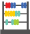Let's learn!

What does regroup mean?
In math, regrouping can be defined as the process of making groups of tens when carrying out operations like addition and subtraction with two-digit numbers or larger.
To regroup means to rearrange groups in place value to carry out an operation.

We use regrouping in subtraction, when digits in the minuend are smaller than the digits in the same place in the subtrahend.

Here’s how we regroup hundred and tens to subtract 182 from 427.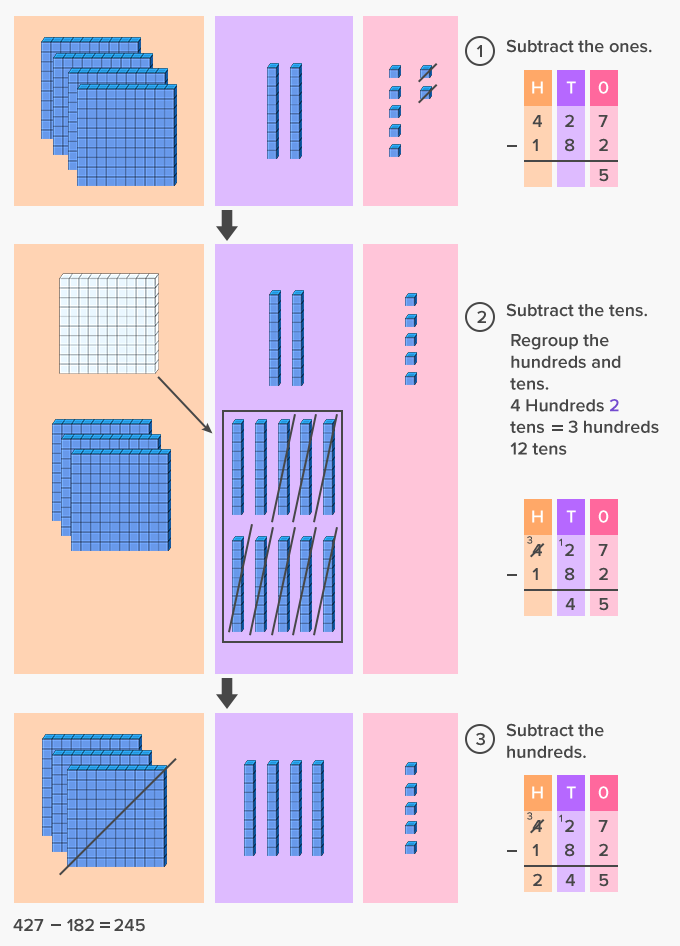We use regrouping in addition when the sum of two digits in the place value column is greater than nine.

Here’s how we regroup ones and tens to add 248 and 75.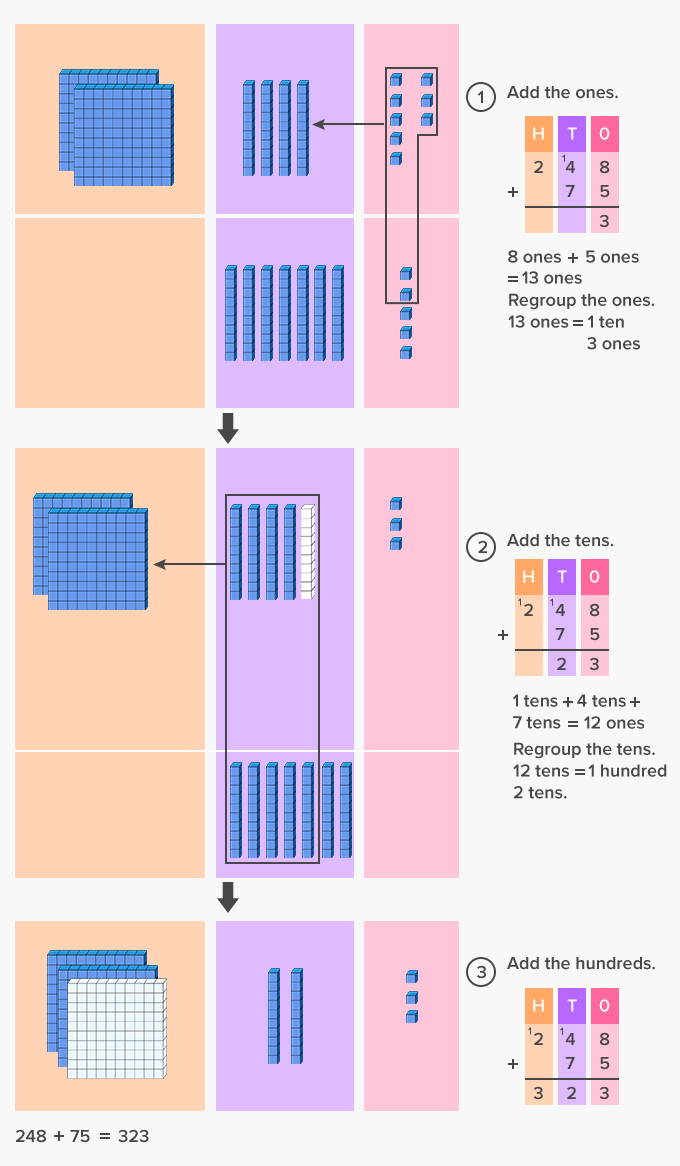Fun Facts Regrouping is referred to as the “carrying forward” in addition and “borrowing” in subtraction problems.

##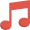Let's sing!

It’s time to trade with ten or more.
Give some or borrow ten from next door.
Then, add or subtract, and you will score!

##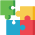Let's do it!

To explain the concept of regrouping in addition and subtraction better, begin by introducing the concept using tens and ones blocks to show how to regroup 10 ones as 1 ten.
You can then ask your child to regroup to add or subtract two- or three-digit numbers using the blocks.

##Related math vocabulary

Won Numerous Awards & Honors